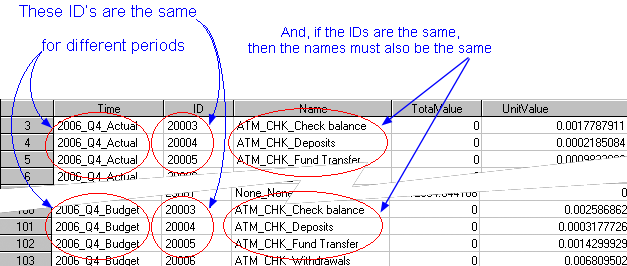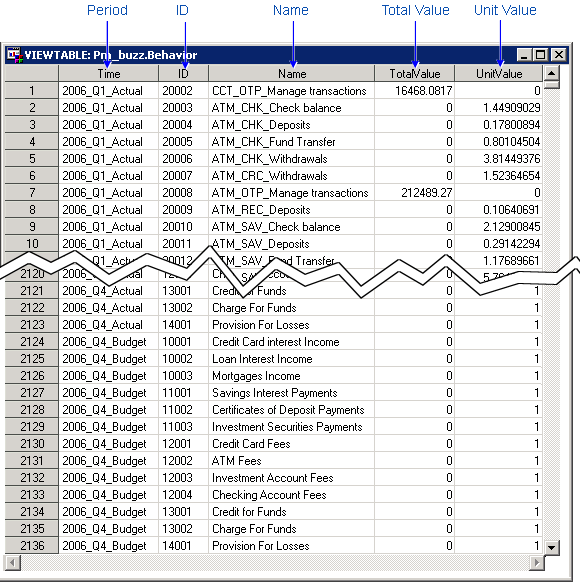Behavior Table

The behavior table lists each source item, with its value, of a transaction cost. Each row in a behavior table:

• represents the lowest level of drill-down in an OLAP view — so, you should not create more behaviors than you expect to view.
• is assigned to a transaction by a rule — so, you should create only as many behaviors as you have rules for assignment.
• must be represented in the hierarchy table. The behavior table represents the leaf nodes of the hierarchy. The hiearchy table defines the parent nodes.

Note: The following message received during cube generation "ERROR: More unique keys were detected in the fact table than in the dimension table for the <hierarchy> dimension" indicates that some behavior table rows are missing from the hierarchy dimension.

A behavior table contains the following columns with a maximum field length as noted:

 Position Name Maximum Field Length Description 1 Time Char 32 The period for the transaction costs 2 ID Char 32 The identifying reference for the behavior 3 Name Char 64 The name of the behavior 4 TotalValue Numeric 8 The total source amount that will be spread 5 UnitValue Numeric 8 The unit cost for each transaction with this source

A behavior table must conform to the following rules:

• Only one of the Unit Value and Total Value columns can contain a non-zero value for any row of the behavior table.
• Each column must have the field length shown.
• The order of the columns is arbitrary (you identify the columns during model definition)
• The name of the column is arbitrary (but if you use these names, the columns are mapped automatically)
• Different behaviors can have the same ID. This is frequently the case when the behaviors represent different time periods. Because rules are attached to behaviors by the behavior ID, having identical IDs allows you to attach the same rule to behaviors from different time periods.

Note: If two behaviors have the same ID, then they must have the same name.

The following picture shows an example of behaviors with the same ID.The following picture shows a sample behavior table: